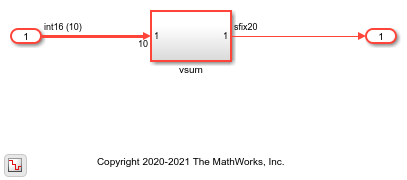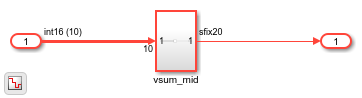# Scalarization of Vector Ports in Generated VHDL Code

This example shows how to flatten the vector signals in a Simulink® model into a structure of scalar signals in the generated VHDL code.

### Specify Scalarization of Vector Ports

When you generate Verilog code, by default, the vector signals are flattened into scalars by default. When you generate VHDL code, you can specify whether to flatten the vector signals on the entire model or at the DUT level. Flattening the vector ports only at the DUT level speeds up code generation especially for large models that have many vector inputs.

You can scalarize the vector ports into scalars when generating HDL code from MATLAB® and Simulink. For the MATLAB® to HDL workflow, in the HDL Code Generation task, on the Clocks & Ports tab, set Scalarize ports to `dutlevel` or `on`.

To scalarize the vector ports when generating HDL code for a Simulink model:

• In the Configuration Parameters dialog box, on the HDL Code Generation > Global Settings > Ports* tab, set Scalarize Ports parameter to `dutlevel` or `on`.

• In the HDL Workflow Advisor, on the HDL Code Generation > Set Code Generation Options > Set Advanced Options > Ports tab, set Scalarize Ports parameter to `dutlevel` or `on`.

• At the MATLAB command prompt, set the `ScalarizePorts` property to `on` or `dutlevel` by using `hdlset_param` or `makehdl`.

See Scalarize ports.

### Vector Sum Model

To see the flattening of vector ports, open the model `hdlcoder_hdlcoder_vector_sum_nested`. The model is driven by a vector input of width `10` and has a scalar output.

```open_system('hdlcoder_vector_sum_nested') set_param('hdlcoder_vector_sum_nested','SimulationCommand','update') ```This model is the same as the `simplevectorsum` model and consists of a Subsystem, `vsum_mid`, inside the `vsum` subsystem.

```open_system('hdlcoder_vector_sum_nested/vsum') ```The `vsum_mid` subsystem contains a Sum of Elements block that is configured for vector summation. The model is configured to use the `Tree` implementation when generating HDL code for the Sum of Elements block within the |vsum subsystem. This implementation is optimized for minimal latency, generates a tree-shaped structure of adders for the block.

```open_system('hdlcoder_vector_sum_nested/vsum/vsum_mid') ```### Scalarize Vector Ports

By default, the `ScalarizePorts` property is `off`. HDL Coder™ generates a type definition and port declaration for the vector port `In1` as shown in this code.

```PACKAGE simplevectorsum_pkg IS TYPE vector_of_std_logic_vector16 IS ARRAY (NATURAL RANGE <>) OF std_logic_vector(15 DOWNTO 0); TYPE vector_of_signed16 IS ARRAY (NATURAL RANGE <>) OF signed(15 DOWNTO 0); END simplevectorsum_pkg; ```

...

```ENTITY vsum IS PORT( In1 : IN vector_of_std_logic_vector16(0 TO 9); -- int16  Out1 : OUT std_logic_vector(19 DOWNTO 0) -- sfix20 ); END vsum; ```

To scalarize the vector ports when generating HDL code, either set the Scalarize ports parameter in the Configuration Parameters dialog box or set the `ScalarizePorts` property to `on` or `dutlevel` with `hdlset_param` or `makehdl`. When you set `ScalarizePorts` to `dutlevel`, only the vector signals at the DUT are flattened into scalars. The scalars are input to the `vsim_mid` subsystem as vectors.

```makehdl('hdlcoder_vector_sum_nested/vsum', 'ScalarizePorts', 'dutlevel') ```
```ENTITY vsum IS PORT( In1_0 : IN std_logic_vector(15 DOWNTO 0); -- int16 In1_1 : IN std_logic_vector(15 DOWNTO 0); -- int16 In1_2 : IN std_logic_vector(15 DOWNTO 0); -- int16 In1_3 : IN std_logic_vector(15 DOWNTO 0); -- int16 In1_4 : IN std_logic_vector(15 DOWNTO 0); -- int16 In1_5 : IN std_logic_vector(15 DOWNTO 0); -- int16 In1_6 : IN std_logic_vector(15 DOWNTO 0); -- int16 In1_7 : IN std_logic_vector(15 DOWNTO 0); -- int16 In1_8 : IN std_logic_vector(15 DOWNTO 0); -- int16 In1_9 : IN std_logic_vector(15 DOWNTO 0); -- int16 Out1 : OUT std_logic_vector(19 DOWNTO 0) -- sfix20 ); END vsum; ```
```ENTITY vsum_mid IS PORT( In1 : IN vector_of_std_logic_vector16(0 TO 9); -- int16  Out1 : OUT std_logic_vector(19 DOWNTO 0) -- sfix20 ); END vsum_mid; ```

To flatten the vector ports on the entire model, set `ScalarizePorts` to `on`. The vector ports at `vsum_mid` and the inputs to the Sum of Elements block are also flattened into scalars.

```makehdl('hdlcoder_vector_sum_nested/vsum', 'ScalarizePorts', 'on') ```
```ENTITY vsum_mid IS PORT( In1_0 : IN std_logic_vector(15 DOWNTO 0); -- int16 In1_1 : IN std_logic_vector(15 DOWNTO 0); -- int16 In1_2 : IN std_logic_vector(15 DOWNTO 0); -- int16 In1_3 : IN std_logic_vector(15 DOWNTO 0); -- int16 In1_4 : IN std_logic_vector(15 DOWNTO 0); -- int16 In1_5 : IN std_logic_vector(15 DOWNTO 0); -- int16 In1_6 : IN std_logic_vector(15 DOWNTO 0); -- int16 In1_7 : IN std_logic_vector(15 DOWNTO 0); -- int16 In1_8 : IN std_logic_vector(15 DOWNTO 0); -- int16 In1_9 : IN std_logic_vector(15 DOWNTO 0); -- int16 Out1 : OUT std_logic_vector(19 DOWNTO 0) -- sfix20 ); END vsum_mid; ```

### Usage Notes and Restrictions

• When you use the `ScalarizePorts` property for a protected model, you must use the same value for this property as the value specified for this parameter in the top model from which it is referenced.

• When you use the `IP Core Generation` and `FPGA-in-the-Loop` workflows, vector ports are not supported. Set `ScalarizePorts` as `on` or `dutlevel`. For faster code generation, set `ScalarizePorts` to `dutlevel`.

• Vector or matrix ports as input to a model reference interface must be scalarized before generating HDL code. Set `ScalarizePorts` to `dutlevel`.

• By default, Generate VHDL code for model references into a single library is enabled. The VHDL code is generated in a single library instead of separate libraries. In this case, set the `ScalarizePorts` property to `off` before generating HDL code.

• When generating code, if you encounter typing or naming conflicts between vector ports when interfacing two or more generated VHDL code modules, use the `ScalarizePorts` property to generate non-conflicting port definitions.